V.5 No 2 33 On basic formalism of special theory of relativity4. On reality of relativistic reduction of space-time Before, in works analysing Doppler effect  and aberration , we not once showed erroneous the relativistic interpretation of Lorentz reduction, in particular their groundless answer to the question, whether the Lorentz reduction is real. They think, “doubt usually arises because the length of moving bodies is determined arbitrarily in that measure as the conventional definition of simultaneity is arbitrary (e.g., Einsteinian which we use). But we can decisively answer, this phenomenon is real, as one and the same procedure of length measurement will give different results with classical (Newtonian) theory and theory of relativity” [12, p. 64]. But the issue is not the fact of length measurement. Finiteness of light speed has been proven in classical theory, as well as non-simultaneity of light rays arriving from the ends of measurement rod also has been studied in classical formalism by Poincare, Lorentz and many other authors. The point is not, how much the non-simultaneity revealed in measurements will result the real reduction of the very object. The rays will not arrive to the observer because of the object’s reduction. Anyway there will be difference in rays arriving from the ends of measured rod. On the other hand, which concern can have the finiteness of light speed to the reduction of object? To clear this issue, let us analyse the features of relativistic conception from the view of self-consistence of its formalism, which has to satisfy the wholeness of the transformation group. “Every possible theory based on measurement premises to introduce the class of reference frame systems, in which the device is oriented. Therefore, each theory, as far as it is theory, not an amount of incoherent facts, includes as a necessary premise preceding the experiment some principle on whose grounds the measured results are recalculated from one reference frame to another. In other words, the reference necessarily premises the criterion of equality, which is understood as the relativity principle, i.e. as the statement of independence of measurement results with respect to a definite class of coordinate transformation that form a group. The fact that an arbitrary class of such transformations actually forms a group follows from the notice of Weyl that the known axioms of equality - transitivity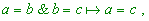(50)
 reflexivity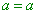(51)
 and symmetry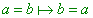(52)
 - transit into group axioms, if the relation of equality is introduced by an arbitrary mapping of some set into itself (automorphism) (i.e. in the way we used in the previous part of our study, when analysed the law of vectors summation - Authors)” [13, p. 348]. ”But such understanding of systematics premises the related group to be intransitive, i.e. invariants have to exist. The transitive group that does not remain invariant any properties of studied objects in which the classification is made identifies in this way (! - Authors) all elements of the set {f}, leading to degeneration of systematics and, consequently, the structure” [ibidem, p. 349]. This definition concerns, of course, Lorentz transformations, the more that ”geometry of Minkowski space is the geometry of the group of Lorentz automorphisms” [14, p. 94]. ”By virtue of equivalent coordinates x1, x2 , x3 , the transformation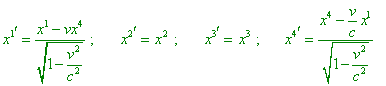(53)
 assumes two analogues more. Besides, it is obvious that trivial turns and transfers (at x4 = x4' ) do not change the form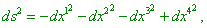(54)
 as well as the time shift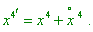Therefore, there exists a group of linear real transformations being the group of automorphism of Minkowski space that depends on 10 arbitrary parameters; it includes 3 Lorentz turnings, 3 spatial turnings and 4 shifts in axes. It is well known … that the number of independent parameters in motion, 10, is maximal for any V4 . With it we abstract from mirror reflections like xa = xa'   , which also retain (54) to be invariant and increase the number of different transformations” [14, p. 94]. However, with all above complete substantiation of wholeness of group of Lorentz transformations, Petrov has omitted an important detail concerning not the very Lorentz transformation (53) but the transformation secondary to this first - above we made sure that namely in secondary transformations there is an inner inconsistency. But Lorentz transformations as such, in the meaning of coordinate transformations, are unable to cover the whole versatility of kinematic and dynamic problems of systems. So, to make sure that the inner consistence of the group of automorphisms of Lorentz transformation is complete, we have additionally to analyse the completeness of secondary transformations. To begin, let us check as an example, how Lorentz transformations for coordinates satisfy the condition of transitiveness (50). For it, let us give three identical inertial frames K1, K2, K3 whose axes x1, x2, x3  will be coincided, in order to simplify, and the frames K2  and K3  are moving with respect to K1 with different speeds. Furthermore, we will suppose that K2   moves with respect to K1  with the speed v12 , and K3  moves with respect to K2 with the speed v23 . Now we can make use of condition that the calculation has to be independent of the way of calculation, then we will sequentially determine the coordinate transformation from K1  to K3  through K2 . Then in accordance with (53),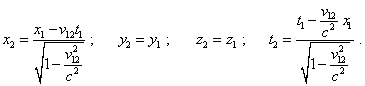(55)
 In its turn,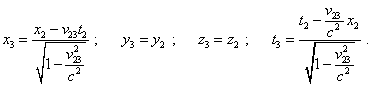(56)
 Substituting (55) into (56), we yield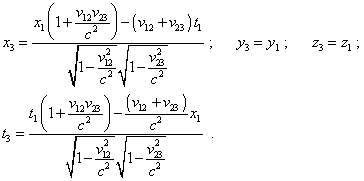(57)
 Then, to make a direct transformation from K1   to K3 , avoiding K2 , we have to determine the speed v13 . For it, we will use the theorem of relativistic speed summation, and yield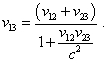(58)
 Now, substituting (58) into equations of standard Lorentz transformation, yield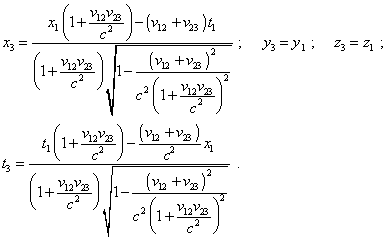(59)
 It is easy to see in a simple algebraic transformation that the denominators of expressions (59) are identical to those of (57). Thus, we generally proved that Lorentz transformation for coordinates satisfy the transitivity principle. Similarly, we can make sure, these transformations remain true for the rest terms of the group.

Contents: / 29 / 30 / 31 / 32 / 33 / 34 / 35 / 36 / 37 / 38 / 39 /